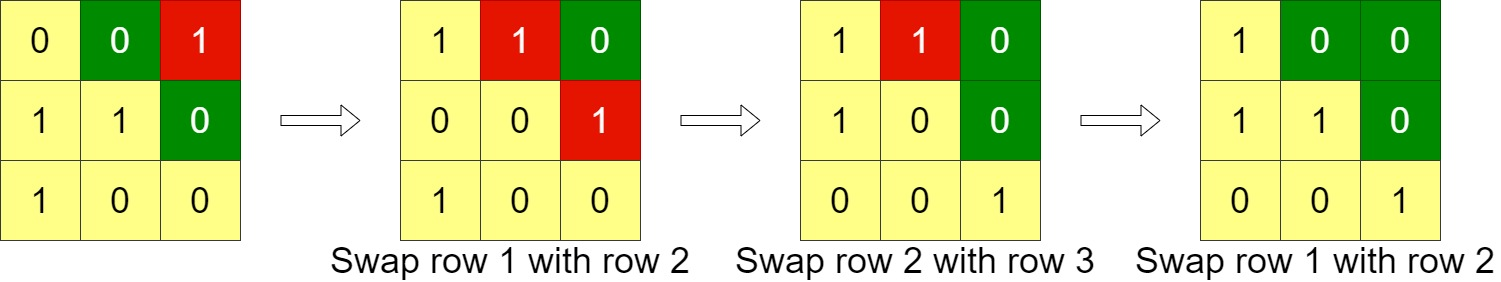##### Welcome to Subscribe On Youtube

Formatted question description: https://leetcode.ca/all/1536.html

# 1536. Minimum Swaps to Arrange a Binary Grid (Medium)

Given an n x n binary grid, in one step you can choose two adjacent rows of the grid and swap them.

A grid is said to be valid if all the cells above the main diagonal are zeros.

Return the minimum number of steps needed to make the grid valid, or -1 if the grid cannot be valid.

The main diagonal of a grid is the diagonal that starts at cell (1, 1) and ends at cell (n, n).

Example 1:Input: grid = [[0,0,1],[1,1,0],[1,0,0]]
Output: 3


Example 2:Input: grid = [[0,1,1,0],[0,1,1,0],[0,1,1,0],[0,1,1,0]]
Output: -1
Explanation: All rows are similar, swaps have no effect on the grid.


Example 3:Input: grid = [[1,0,0],[1,1,0],[1,1,1]]
Output: 0


Constraints:

• n == grid.length
• n == grid[i].length
• 1 <= n <= 200
• grid[i][j] is 0 or 1

Related Topics:
Greedy

## Solution 1.

First store the max index of the 1 in each row into a vector<int> v.

Then we can from the first item to the last item in v:

• if v[i] <= i, this row is good. Skip
• Otherwise, we find the minimal j (i + 1 < j < N) that satisfies v[j] <= i.
• If we can’t find it, no row can be placed at this i-th row. Return -1.
• Otherwise, we move v[j] to v[i] and the v[k] (i <= k < j) are moved downwards. And we add j - i steps of swaps to the answer.
// https://leetcode.com/problems/minimum-swaps-to-arrange-a-binary-grid/
// Time: O(N^2)
// Space: O(N)
class Solution {
public:
int minSwaps(vector<vector<int>>& G) {
int N = G.size(), ans = 0;
vector<int> v(N);
for (int i = 0; i < N; ++i) {
int len = 0;
for (int j = N - 1; j >= 0; --j) {
if (G[i][j] == 0) continue;
len = j + 1;
break;
}
v[i] = len;
}
for (int i = 0; i < N; ++i) {
if (v[i] <= i + 1) continue;
int j = i + 1;
while (j < N && v[j] > i + 1) ++j;
if (j == N) return -1;
int tmp = v[j];
for (int k = j; k > i; --k) v[k] = v[k - 1];
v[i] = tmp;
ans += j - i;
}
return ans;
}
};

• class Solution {
public int minSwaps(int[][] grid) {
int side = grid.length;
int[] zerosCounts = new int[side];
for (int i = 0; i < side; i++) {
int[] row = grid[i];
int zerosCount = 0;
for (int j = side - 1; j >= 0; j--) {
if (row[j] == 0)
zerosCount++;
else
break;
}
zerosCounts[i] = zerosCount;
}
int swaps = 0;
for (int i = 0; i < side; i++) {
int minZeros = side - i - 1;
if (zerosCounts[i] < minZeros) {
int firstRowIndex = -1;
for (int j = i + 1; j < side; j++) {
if (zerosCounts[j] >= minZeros) {
firstRowIndex = j;
break;
}
}
if (firstRowIndex < 0)
return -1;
for (int j = firstRowIndex; j > i; j--) {
swaps++;
int temp = zerosCounts[j];
zerosCounts[j] = zerosCounts[j - 1];
zerosCounts[j - 1] = temp;
}
}
}
return swaps;
}
}

• // https://leetcode.com/problems/minimum-swaps-to-arrange-a-binary-grid/
// Time: O(N^2)
// Space: O(N)
class Solution {
public:
int minSwaps(vector<vector<int>>& G) {
int N = G.size(), ans = 0;
vector<int> v(N);
for (int i = 0; i < N; ++i) {
int len = 0;
for (int j = N - 1; j >= 0; --j) {
if (G[i][j] == 0) continue;
len = j + 1;
break;
}
v[i] = len;
}
for (int i = 0; i < N; ++i) {
if (v[i] <= i + 1) continue;
int j = i + 1;
while (j < N && v[j] > i + 1) ++j;
if (j == N) return -1;
int tmp = v[j];
for (int k = j; k > i; --k) v[k] = v[k - 1];
v[i] = tmp;
ans += j - i;
}
return ans;
}
};